# Extended Mo’s Algorithm with ≈ O(1) time complexity

• Difficulty Level : Hard
• Last Updated : 19 Jan, 2022

Given an array of n elements and q range queries (range sum in this article) with no updates, task is to answer these queries with efficient time and space complexity. The time complexity of a range query after applying square root decomposition comes out to be O(√n). This square-root factor can be decreased to a constant linear factor by applying square root decomposition on the block of the array which was decomposed earlier.
Prerequisite: Mo’s Algorithm | Prefix Array

Approach :
As we apply square root decomposition to the given array, querying a range-sum comes in O(√n) time.
Here, calculate the sum of blocks which are in between the blocks under consideration(corner blocks), which takes O(√n) iterations.
Initial Array :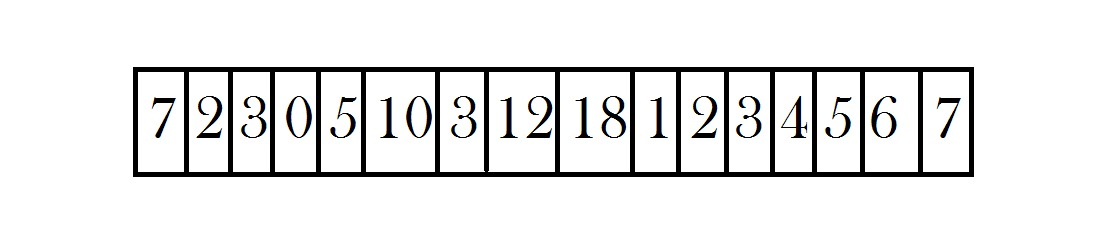Initial Array

Decomposition of array into blocks :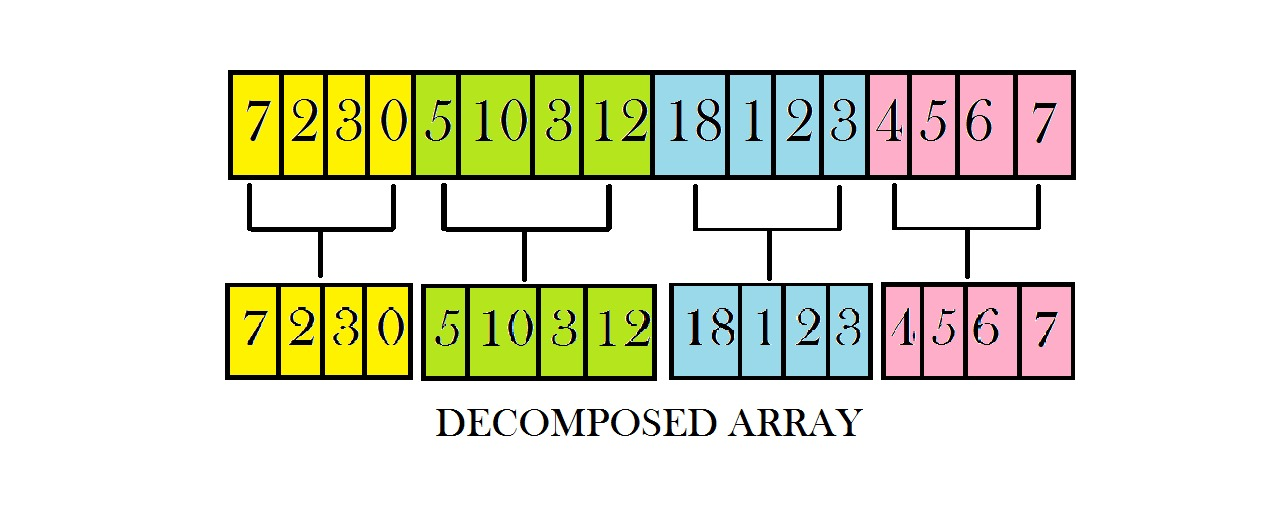Decomposition at level-1

And the calculation time for the sum on the starting block and ending block both takes O(√n) iterations.

Which will leaves us per query time complexity of :

```= O(√n) + O(√n) + O(√n)
= 3 * O(√n)
~O(√n)```

Here, we can reduce the runtime complexity of our query algorithm cleverly by calculating blockwise prefix sum and using it to calculate the sum accumulated in the blocks which lie between the blocks under consideration. Consider the code below :

`interblock_sum[x1][x2] = prefixData[x2 - 1] - prefixData[x1];`

Time taken for calculation of above table is :

```= O(√n) * O(√n)
~O(n)```

NOTE : We haven’t taken the sum of blocks x1 & x2 under consideration as they might be carrying partial data.
Prefix Array :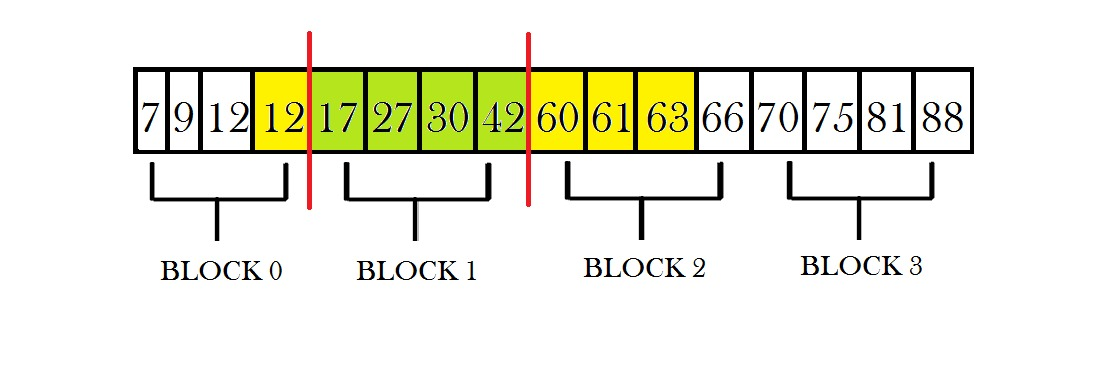Prefix Array

Suppose we want to query for the sum for range from 4 to 11, we consider the sum between block 0 and block 2 (excluding the data contained in block 0 and block 1), which can be calculated using the sum in the green coloured blocks represented in the above image.
Sum between block 0 and block 2 = 42 – 12 = 30
For calculation of rest of the sum present in the yellow blocks, consider the prefix array at the decomposition level-2 and repeat the process again.
Here, observe that we have reduced our time complexity per query significantly, though our runtime remains similar to our last approach :

Our new time complexity can be calculated as :

```= O(√n) + O(1) + O(√n)
= 2 * O(√n)
~O(√n)    ```

Square-root Decomposition at Level-2 :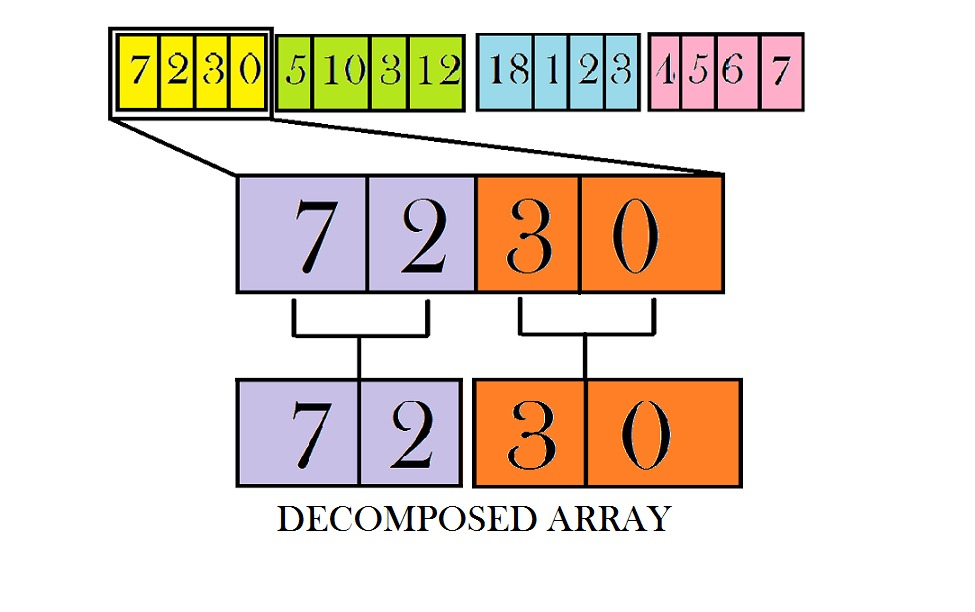Decomposition at level-2

Further, we apply square root decomposition again on every decomposed block retained from the previous decomposition. Now at this level, we have approximately √√n sub-blocks in each block which were decomposed at last level. So, we need to run a range query on these blocks only two times, one time for starting block and one time for ending block.

Precalculation Time taken for level 2 decomposition :
No of blocks at level 1 ~ √n
No of blocks at level 2 ~ √√n

Level-2 Decomposition Runtime of a level-1 decomposed block :

`= O(√n)`

Overall runtime of level-2 decomposition over all blocks :

```= O(√n) * O(√n)
~O(n)```

Now, we can query our level-2 decomposed blocks in O(√√n) time.
So, we have reduced our overall time complexity from O(√n) to O(√√n)

Time complexity taken in querying edge blocks :

```= O(√√n) + O(1) + O(√√n)
= 2 * O(√√n)
~O(√√n)```

Total Time complexity can be calculated as :

```= O(√√n)+O(1)+O(√√n)
= 2 * O(√√n)
~O(√√n)  ```

Square-root Decomposition at Level-3 :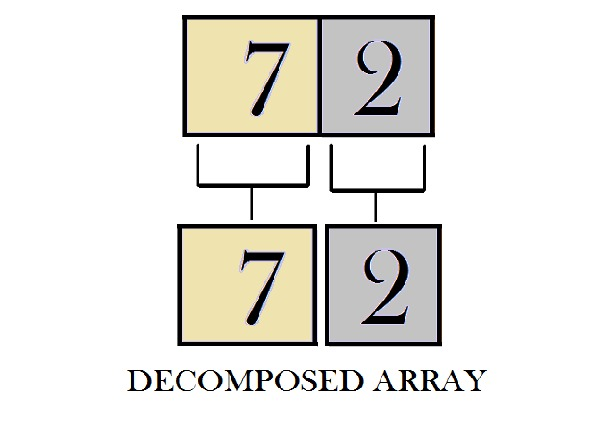Decomposition at level-3

Using this method we can decompose our array again and again recursively d times to reduce our time complexity to a factor of constant linearity.

`O(d * n1/(2^d)) ~ O(k), as d increases this factor converges to a constant linear term`

The code presented below is a representation of triple square root decomposition where d = 3:

`O(q * d * n1/(2 ^ 3)) ~ O(q * k) ~ O(q) `

[where q represents number of range queries]

## C++

 `// CPP code for offline queries in``// approx constant time.``#include``using` `namespace` `std;` `int` `n1;` `// Structure to store decomposed data``typedef` `struct``{``    ``vector<``int``> data;``    ``vector> rdata;``    ``int` `blocks;``    ``int` `blk_sz;``}sqrtD;` `vector> Sq3;``vector Sq2;``sqrtD Sq1;` `// Square root Decomposition of``// a given array``sqrtD decompose(vector<``int``> arr)``{``    ``sqrtD sq;``    ``int` `n = arr.size();``    ``int` `blk_idx = -1;``    ``sq.blk_sz = ``sqrt``(n);``    ``sq.data.resize((n/sq.blk_sz) + 1, 0);``    ` `    ``// Calculation of data in blocks``    ``for` `(``int` `i = 0; i < n; i++)``    ``{``        ``if` `(i % sq.blk_sz == 0)``        ``{``            ``blk_idx++;``        ``}``        ``sq.data[blk_idx] += arr[i];``    ``}``    ` `    ``int` `blocks = blk_idx + 1;``    ``sq.blocks = blocks;``    ` `    ``// Calculation of prefix data``    ``int` `prefixData[blocks];``    ``prefixData = sq.data;``    ``for``(``int` `i = 1; i < blocks; i++)``    ``{``        ``prefixData[i] =``          ``prefixData[i - 1] + sq.data[i];``    ``}``    ` `    ``sq.rdata.resize(blocks + 1,``             ``vector<``int``>(blocks + 1));``    ` `    ``// Calculation of data between blocks``    ``for``(``int` `i = 0 ;i < blocks; i++)``    ``{``        ``for``(``int` `j = i + 1; j < blocks; j++)``        ``{``            ``sq.rdata[i][j] = sq.rdata[j][i] =``            ``prefixData[j - 1] - prefixData[i];``        ``}``    ``}``    ` `    ``return` `sq;``}` `// Square root Decomposition at level3``vector> tripleDecompose(sqrtD sq1,``                      ``sqrtD sq2,vector<``int``> &arr)``{``    ``vector> sq(sq1.blocks,``                      ``vector(sq1.blocks));``    ` `    ``int` `blk_idx1 = -1;``    ` `    ``for``(``int` `i = 0; i < sq1.blocks; i++)``    ``{``        ``int` `blk_ldx1 = blk_idx1 + 1;``        ``blk_idx1 = (i + 1) * sq1.blk_sz - 1;``        ``blk_idx1 = min(blk_idx1,n1 - 1);` `        ``int` `blk_idx2 = blk_ldx1 - 1;``        ` `        ``for``(``int` `j = 0; j < sq2.blocks; ++j)``        ``{``            ``int` `blk_ldx2 = blk_idx2 + 1;``            ``blk_idx2 = blk_ldx1 + (j + 1) *``                       ``sq2.blk_sz - 1;``            ``blk_idx2 = min(blk_idx2, blk_idx1);``        ` `            ``vector<``int``> ::iterator it1 =``                        ``arr.begin() + blk_ldx2;``            ``vector<``int``> ::iterator it2 =``                        ``arr.begin() + blk_idx2 + 1;``            ``vector<``int``> vec(it1, it2);``            ``sq[i][j] = decompose(vec);    ``        ``}``    ``}``    ``return` `sq;        ``}` `// Square root Decomposition at level2``vector doubleDecompose(sqrtD sq1,``                              ``vector<``int``> &arr)``{``    ``vector sq(sq1.blocks);``    ``int` `blk_idx = -1;``    ``for``(``int` `i = 0; i < sq1.blocks; i++)``    ``{``        ``int` `blk_ldx = blk_idx + 1;``        ``blk_idx = (i + 1) * sq1.blk_sz - 1;``        ``blk_idx = min(blk_idx, n1 - 1);``        ``vector<``int``> ::iterator it1 =``                    ``arr.begin() + blk_ldx;``        ``vector<``int``> ::iterator it2 =``                    ``arr.begin() + blk_idx + 1;``        ``vector<``int``> vec(it1, it2);``        ``sq[i] = decompose(vec);``    ``}``    ` `    ``return` `sq;    ``}` `// Square root Decomposition at level1``void` `singleDecompose(vector<``int``> &arr)``{``    ``sqrtD sq1 = decompose(arr);``    ``vector sq2(sq1.blocks);``    ``sq2 = doubleDecompose(sq1, arr);``    ` `    ``vector> sq3(sq1.blocks,``           ``vector(sq2.blocks));``           ` `    ``sq3 = tripleDecompose(sq1, sq2,arr);``        ` `    ``// ASSIGNMENT TO GLOBAL VARIABLES``    ``Sq1 = sq1;``    ``Sq2.resize(sq1.blocks);``    ``Sq2 = sq2;``    ``Sq3.resize(sq1.blocks,``        ``vector(sq2.blocks));``    ``Sq3 = sq3;``}` `// Function for query at level 3``int` `queryLevel3(``int` `start,``int` `end, ``int` `main_blk,``                ``int` `sub_main_blk, vector<``int``> &arr)``{``    ``int` `blk_sz= Sq3.blk_sz;` `    ``// Element Indexing at level2 decomposition``    ``int` `nstart = start - main_blk *``        ``Sq1.blk_sz - sub_main_blk * Sq2.blk_sz;``    ``int` `nend = end - main_blk *``        ``Sq1.blk_sz - sub_main_blk * Sq2.blk_sz;` `    ``// Block indexing at level3 decomposition``    ``int` `st_blk = nstart / blk_sz;``    ``int` `en_blk = nend / blk_sz;``    ` `    ``int` `answer =``        ``Sq3[main_blk][sub_main_blk].rdata[st_blk][en_blk];``    ` `    ``// If start and end point don't lie in same block``    ``if``(st_blk != en_blk)``    ``{``        ``int` `left = 0, en_idx = main_blk * Sq1.blk_sz +``                      ``sub_main_blk * Sq2.blk_sz +``                      ``(st_blk + 1) * blk_sz -1;``    ` `        ``for``(``int` `i = start; i <= en_idx; i++)``        ``{``            ``left += arr[i];``        ``}``        ` `        ``int` `right = 0, st_idx = main_blk * Sq1.blk_sz +``                       ``sub_main_blk * Sq2.blk_sz +``                       ``(en_blk) * blk_sz;``        ` `        ``for``(``int` `i = st_idx; i <= end; i++)``        ``{``            ``right += arr[i];``        ``}``        ` `        ``answer += left;``        ``answer += right;``    ``}``    ``else``    ``{``        ``for``(``int` `i = start; i <= end; i++)``        ``{``            ``answer += arr[i];``    ``}``}``return` `answer;    ``}` `// Function for splitting query to level two``int` `queryLevel2(``int` `start, ``int` `end, ``int` `main_blk,``                ``vector<``int``> &arr)``{``    ``int` `blk_sz = Sq2.blk_sz;` `    ``// Element Indexing at level1 decomposition``    ``int` `nstart = start - (main_blk * Sq1.blk_sz);``    ``int` `nend = end - (main_blk * Sq1.blk_sz);` `    ``// Block indexing at level2 decomposition``    ``int` `st_blk = nstart / blk_sz;``    ``int` `en_blk = nend / blk_sz;``    ` `    ``// Interblock data level2 decomposition``    ``int` `answer = Sq2[main_blk].rdata[st_blk][en_blk];``    ` `    ``if``(st_blk == en_blk)``    ``{``        ``answer += queryLevel3(start, end, main_blk,``                              ``st_blk, arr);``    ``}``    ``else``    ``{``        ``answer += queryLevel3(start, (main_blk *``                  ``Sq1.blk_sz) + ((st_blk + 1) *``                  ``blk_sz) - 1, main_blk, st_blk, arr);``        ` `        ``answer += queryLevel3((main_blk * Sq1.blk_sz) +``                  ``(en_blk * blk_sz), end, main_blk, en_blk, arr);``    ``}``    ``return` `answer;    ``}` `// Function to return answer according to query``int` `Query(``int` `start,``int` `end,vector<``int``>& arr)``{``    ``int` `blk_sz = Sq1.blk_sz;``    ``int` `st_blk = start / blk_sz;``    ``int` `en_blk = end / blk_sz;``    ` `    ``// Interblock data level1 decomposition    ``    ``int` `answer = Sq1.rdata[st_blk][en_blk];` `    ``if``(st_blk == en_blk)``    ``{``        ``answer += queryLevel2(start, end, st_blk, arr);``    ``}``    ``else``    ``{``        ``answer += queryLevel2(start, (st_blk + 1) *``                  ``blk_sz - 1, st_blk, arr);``        ``answer += queryLevel2(en_blk * blk_sz, end,``                              ``en_blk, arr);``    ``}``    ` `    ``// returning final answer``    ``return` `answer;``}` `// Driver code``int` `main()``{    ``    ``n1 = 16;``    ` `    ``vector<``int``> arr = {7, 2, 3, 0, 5, 10, 3, 12,``                       ``18, 1, 2, 3, 4, 5, 6, 7};` `    ``singleDecompose(arr);``    ` `    ``int` `q = 5;``    ``pair<``int``, ``int``> query[q] = {{6, 10}, {7, 12},``                  ``{4, 13}, {4, 11}, {12, 16}};``    ` `    ``for``(``int` `i = 0; i < q; i++)``    ``{``        ``int` `a = query[i].first, b = query[i].second;``        ``printf``(``"%d\n"``, Query(a - 1, b - 1, arr));``    ``}``    ` `    ``return` `0;``}`

Output:

```44
39
58
51
25```

Time Complexity : O(q * d * n1/(2^3)) ≈ O(q * k) ≈ O(q)
Auxiliary Space : O(k * n) ≈ O(n)
Note : This article is to only explain the method of decomposing the square root to further decomposition.

My Personal Notes arrow_drop_up